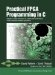# Section 10.3. Refinement 2: Array Splitting

### 10.3. Refinement 2: Array Splitting

One of the most significant performance advantages of hardware implementations is the ability to access multiple memories in the same cycle. In the typical software implementation, each CPU is connected to one or more memories by a single bus. In that scenario, the software can only access data from, at most, one memory per bus transaction (one or more cycles).

When generating hardware, we have the opportunity to generate any topology necessary, including separate connections to multiple memories. The Impulse C tools generate a separate, individually connected memory for each array used in the process. For example, consider the following:

` void run() {     co_int32 i,A,B,C;    for (i=0; i<4; i++) {       A[i]=B[i]+C[i];    } `

This process generates three separate memories for the arrays A, B, and C, each with a dedicated connection to the computation hardware. The assignment statement that would require at least three bus transactions to execute in software can now be performed in a single cycle by performing reads from B and C and writing to A simultaneously.

Returning to our DES example, notice that two relatively large arrays are involved in the core computation, Spbox and Ks. These are each implemented in their own memory, but they still involve multiple accesses to the same array and thus require multiple cycles when used in this algorithm. Let's look at the memory access involved in the core computation as specified in macro F and in the main code loop:

` #define F(l,r,key){\    work = ((r >> 4) | (r << 28)) ^ key;\    l ^= Spbox[work & 0x3f];\    l ^= Spbox[(work >> 8) & 0x3f];\    l ^= Spbox[(work >> 16) & 0x3f];\    l ^= Spbox[(work >> 24) & 0x3f];\    work = r ^ key;\    l ^= Spbox[work & 0x3f];\    l ^= Spbox[(work >> 8) & 0x3f];\    l ^= Spbox[(work >> 16) & 0x3f];\    l ^= Spbox[(work >> 24) & 0x3f];\  } ...   for (i=0; i<16; i++) {          F(left,right,Ks[i]);       i++;       F(right,left,Ks[i]); } `

Each iteration of this loop requires four loads from Ks and eight loads from Spbox. The need for eight loads from Spbox implies that at least eight cycles will be required because (at most) one value can be read from Spbox per cycle. Notice, however, that Spbox is a multidimensional array and that the row indices are all constants. Because the indices are constant it is possible to actually split this array into individual arrays for each row.

Likewise, notice that Ks is a multidimensional array and the column indices are all constant. Ks can therefore be split into individual arrays for each column. The resulting code looks like this:

` #define F(l,r,key0,key1){\    work = ((r >> 4) | (r << 28)) ^ key0;\    l ^= Spbox6[work & 0x3f];\    l ^= Spbox4[(work >> 8) & 0x3f];\    l ^= Spbox2[(work >> 16) & 0x3f];\    l ^= Spbox0[(work >> 24) & 0x3f];\    work = r ^ key1;\    l ^= Spbox7[work & 0x3f];\    l ^= Spbox5[(work >> 8) & 0x3f];\    l ^= Spbox3[(work >> 16) & 0x3f];\    l ^= Spbox1[(work >> 24) & 0x3f];\  }  ...   i=0;   do {       F(left,right,Ks0[i],Ks1[i]);      i++;      F(right,left,Ks0[i],Ks1[i]);      i++;   }while (i<16); `

This is only a minor change to the code, but the result is that each of the new Spbox and Ks arrays are accessed only twice per iteration, reducing the total cycle count required to execute the process. After regenerating the hardware with CoDeveloper, we obtain an implementation that is slightly smaller in size and 19.5 times faster than the unmodified software implementation running on MicroBlaze. In this case, array splitting has doubled the performance over our first refinement. The result is also smaller because some address calculations have been eliminated by reducing the dimension of Spbox and Ks.

Tip

Array splitting is a useful technique for reducing both size and cycle counts.Practical FPGA Programming in C
ISBN: 0131543180
EAN: 2147483647
Year: 2005
Pages: 208